Rule (→)  versus  RuleDelayed (:→)

A good application of RuleDelayed (:→) is in the following line.
Here RuleDelayed (:→) must be used instead of Rule (→)
since Factor must not evaluate until it is given the argument of Log.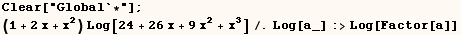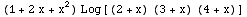The function below will modify any expression so all approximate numbers that
are equal to an integer are converted to the equivalent integer.  In this
case RuleDelayed (:→) must be used instead of Rule (→)
since the rule must not evaluate until it has an actual value to operate
on.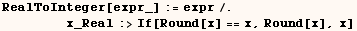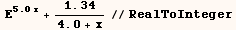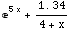Another for using (lhs:→rhs) instead of (lhs→rhs) is when
(lhs) includes named patterns. Named patterns can include (x_), (x__),
(opts___), (m_:4) (j_Integer), (t_?Positive), (n_.) and many other forms. To
demonstrate how use of (lhs→rhs) can cause problems consider the input
in the next cell.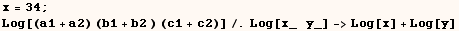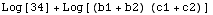In the cell above the global value of (x) was used where we wanted (a1+a2) to
go.  Most often the problem is much more subtle than in the example above.
Typically the variable used to name the pattern had a value assigned long
before you use it in (lhs→rhs). One method sure to avoid this problem
is to clear the values from any variables used to name patterns as in the
next cell. This of course requires that you don't have values assigned to (x)
or (y) that you care about.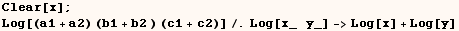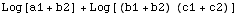Another, often more desirable, method of avoiding the error above is to use
(lhs:→rhs) instead of (lhs→rhs).  This is done in the next
cell, and the value assigned to (x) doesn't prevent us from getting the right
result.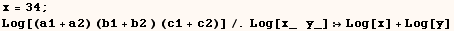The only time when using (lhs:→rhs) might not work very well is
when you can save a lot of time by evaluating (rhs) only once.  An example of
this is given in the next cell where a complicated integral must be evaluated
each time (g2) is used. It's much more efficient to evaluate the integral
only once.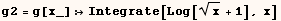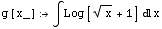In that case you might want to use the next cell where any values assigned  to (x) are cleared, and the integral is evaluated immediately using (lhs →rhs). It's also possible to get the best of both worlds where (rhs)  evaluates immediately as in the next cell, and gives the right result even if  (x) has an assigned value!  To do that you need to use a package I make  available at  http://library.wolfram.com/database/MathSource/425.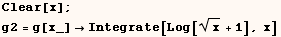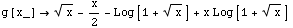Created by Mathematica  (May 16, 2004)

Back to Ted’s Tricks index page